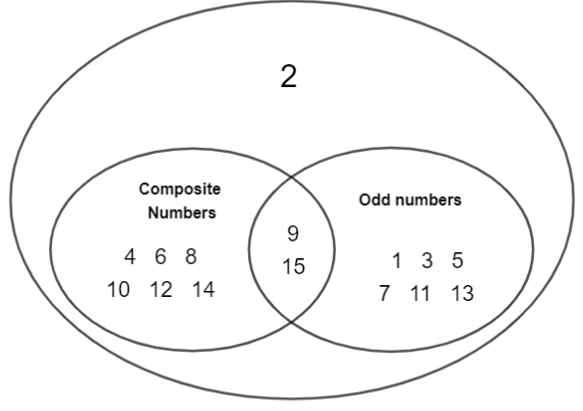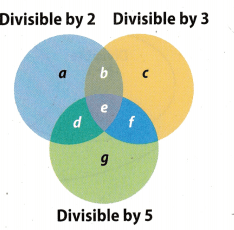# Texas Go Math Grade 7 Lesson 1.2 Answer Key Relationships Between Sets of Rational Numbers

Refer to our Texas Go Math Grade 7 Answer Key Pdf to score good marks in the exams. Test yourself by practicing the problems from Texas Go Math Grade 7 Lesson 1.2 Answer Key Relationships Between Sets of Rational Numbers.

## Texas Go Math Grade 7 Lesson 1.2 Answer Key Relationships Between Sets of Rational Numbers

Classify each number by naming the set or sets to which it belongs.

Question 1.
-8
Integer, rational

Question 2.
-102.55 ……….
Rational

Question 3.
$$\frac{9}{2}$$
Rational

Question 4.
3
Whole, integer, rational.

Reflect

Question 5.
Make a Conjecture Jared said that every prime number is an integer. Do you agree or disagree? Explain.
True. Every prime number is a whole number and every whole number is an integer. We can conclude that prime numbers are a subset of the set of integers.

Question 6.
Tell whether the statement “Some rational numbers are integers” is true or false. Explain your choice.
True Integers are a subset of the set of rationaL numbers. Thus, some rational numbers are integers.

Question 7.
Describe a real-world situation that is best described by the set of rational numbers.
Group of 5 friends go out and stop for a pizza. Pizza has 8 slices and there are 5 of you. Thus, every member of the crew gets $$\frac{8}{5}$$ slices which is equal to 1$$\frac{3}{5}$$ slices.
∴ Sharing a pizza with friends.

Classify each number by naming the set or sets to which it belongs. (Example 1)

Question 1.
5
whole number, __________
whole number, integer, rational number

Question 2.
– $$\frac{3}{14}$$
rational number

Question 3.
-23
integer __________
integer, rational number

Question 4.
4.5
rational number

Tell whether the given statement is true or false. Explain your choice. (Example 2)

Question 5.
All rational numbers are integers. True / False
False.
All, integers are rational numbers, but not all rational numbers are integers.
E.g. 1.3 is a rational number, but not an integer.

Question 6.
Some integers are whole numbers. True / False
True.
Set of whole numbers is a subset of set of integers, which implies that some integers are whole numbers.

Identify the set of numbers that best describes each situation. Explain your choice. (Example 3)

Question 7.
the number of students in a school
The set of best _____________ describes the situation because
The set of whoLe numbers best describes the situation because the school contains a counting number of students. The possible outcomes are 0, 1, 2, 3, 4,… Since it’s impossible to have a negative number of students, or a fraction of a student, the set of whole numbers is the most precise description.

Question 8.
possible points in a certain board game (…-3, -2, -1,0, 1, 2, 3,…….)
The set of ________________________ best describes the situation because
The set of integers best describes the situation because all. possible points in a certain board game are integers.

Points are rational numbers also, but since points cannot be a fraction, the set of integers is the most precise description.

integers, alt possible points in a certain board game are integers Points are rational numbers aLso, but since any point cannot be a fraction, the set of integers is the most precise description.

Essential Question Check-In

Question 9.
How can you represent how the sets of whole numbers, integers, and rational numbers are related to each other?
We can represent the relation using Venn’s diagram.

Classify each number by naming the set or sets to which it belongs.

Question 10.
-9
integer, rational number

Question 11.
7.5
rational number

Question 12.
789
whole number, integer, rational number

Question 13.
5$$\frac{3}{4}$$
rational number

Fill in each Venn diagram with the whole numbers from 1 to 15. Remember that a composite number is a whole number greater than 1 that is not a prime number.

Question 14.
Whole Number from 1 to 15Venn’s diagram solved.

Question 15.
Whole Number from 1 to 15Venn’s diagram solved.

Tell whether the given statement is true or false. Explain your choice.

Question 16.
All rational numbers are whole numbers.
False.
A set of whoLe numbers is a subset of a set of rational numbers, but not vice versa.
E.g. 3 is a rational number, but not a whole number.

Question 17.
All whole numbers are integers.
True.
Every whole number is included in the set of integers.
A set of whole numbers is a subset of the set of integers.

Question 18.
Some whole numbers are negative.
False
Whole numbers are greater or equal to zero, which means they are not negative.

Question 19.
No positive numbers are integers.
False.
A set of whole numbers is a subset of integers. Thus, some integers are positive.

Identify the set of numbers that best describes each situation. Explain your choice.

Question 20.
possible number of miles you can walk in 1 hour
The set of rational numbers best describes the situation.
You can walk any whole number of miles per hour, but you can also walk a fraction of a miLe per hour.

Question 21.
possible number of marbles in a jar
The set of whole numbers best describes the situation.

The jar may contain no marbles or any counting number of marbLes.

The possible numbers of marbLes O, 1, 2, 3,… are whole numbers.

Whole numbers are also integers and rational numbers.

But since there cannot be a negative or a fractional number of bills, the set of whole numbers is the most precise description.

Question 22.
Represent Real-World Problems Using what you know of rational numbers, describe a real-world situation where a doctor might use the set of rational numbers on a daily basis.
A doctor may prescribe 1$$\frac{1}{2}$$ of some kind of pill to a patient

Daily intake of pills.

H.O.T.S Focus on Higher Order Thinking

Question 23.
Communicate Mathematical Ideas The letters in the Venn Divisible by 2 Divisible by 3 diagram represent whole numbers. Describe the numbers you would find in Section c, Section d, and Section e.Section c – Whole numbers divisible by 3, and not divisibLe by 2 or 5
Section d – WhoLe numbers divisible by 2 and 5, and not divisible by 3. Thus, divisible by 10.
Section e – Whole numbers divisible by 2, 3 and 5 Thus, divisible by 30

Question 24.
Analyze Relationships Explain how the set of integers differs from the set of whole numbers.